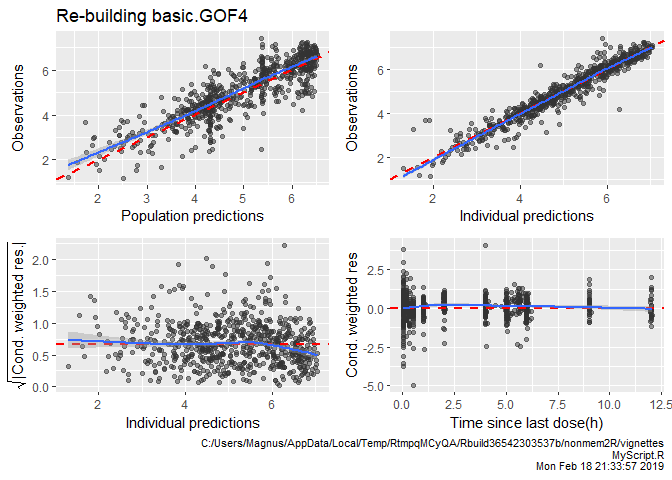# nonmem2R goodness of fit plot functions

## Introduction

Herein is a demo of functions for GOF plots in the nonmem2R R-package. The functions provide building blocks for doing GOF plots with flexibility for user specific formatting and or layout. There are also functions for combining single GOF’s into one and there are basic GOF’s combining a specific type of GOF’s into one single plot, e.g. the function basic.GOF4 which generated the example below.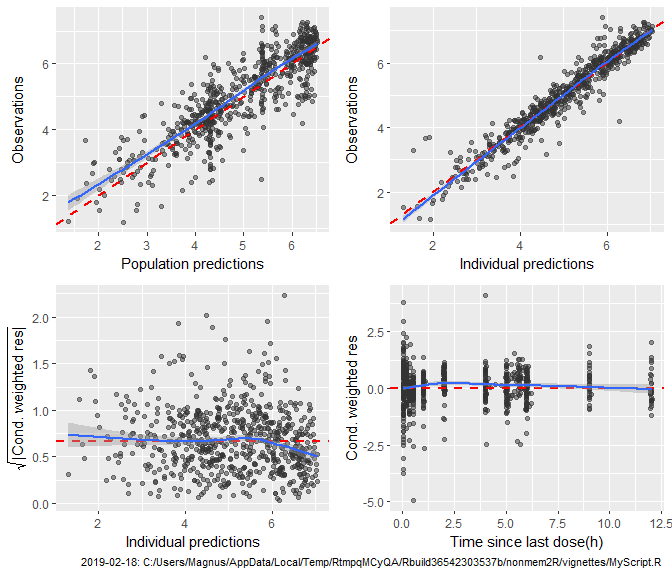The GOF functions build on ggplot2 and as far as possible return an ggplot-object that can be further modified by adding ggplot formatting. However, some functions use grid.arrange from the R-package gridExtra to combine different type of GOF’s. These functions plot the combined set of GOF’s but does not return an ggplot object, and hence the plots can therefore not be easily further modified/formatted. To still provide options to add ggplot formatting these functions have an argument global.ggplot.options which can be used to add extra ggplot functions on each individual GOF before the GOF’s are combined into one graph by grid.arrange.

Formatting of e.g. lines and plot symbols can also be controlled by the argument control and the function GOF.control or using set.GOF.params to affect all GOF’s.

Axis labels are set for most common NONMEM variables, e.g. CWRES is by default labelled “Cond. weighted res” and TIME is by default labelled “Time after first dose(h)”. See examples in section Labels and formatting options with further details on controling axis labels, changing the caption text and other formatting of the GOF plots.

All GOF functions will by default add a caption in the bottom of the graph to indicate date when figure was generated together with the full path to the script. However, this require that the script name has been set using set.script.name, and then the script name will be pasted together with the date and path as derived from getwd. If script name has not been set no caption will be added. Each GOF plot will have a red dashed reference line and blue solid lines are smoother’s representing the actual data.

All functions require a data.frame as input and thus NONMEM output tables, e.g, sdtab, cotab,.., should be loaded prior using the functions, and if using multiple output tables it’s recommenced to just cbind all of them to a single sdtab data.frame.

## Functions

### Tailored GOF functions

There are a set of specific and tailored GOF functions.

do.individual.GOF will provide GOF plots of PRED, IPRED and DV vs TIME (default) with one panel per subject This will generate one page/plot per 20 subjects (default) and therefore this function is best used when exporting to a PDF. The X axis is default set to TIME but e.g. time after last dose (TAPD) or any column in the input data.frame. Below is a small example from 6 subjects.

do.individual.GOF(subset(sdtab,DV>0 & ID<7 & TIME<50))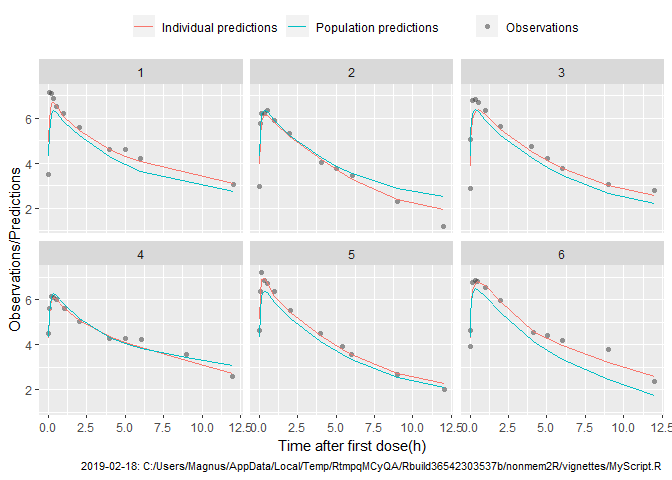On top of basic.GOF4 with an example in the beginning of this document, there is also basic.GOF6 which adds 2 extra GOF plots showing GOF’s for residuals (CWRES) as histogram and as qqnorm plot. Except for number of bins in the histogram of CWRES both have the same options and parameters, e.g. the X-axis value for 1’st and 2’nd GOF on the bottom row is set by idv1 and idv2respectively. In the example below the defult (IPRED and TIME) is replaced by TAPD and PRED.

basic.GOF6(subset(sdtab,DV>0),idv1="TAPD",idv2="PRED")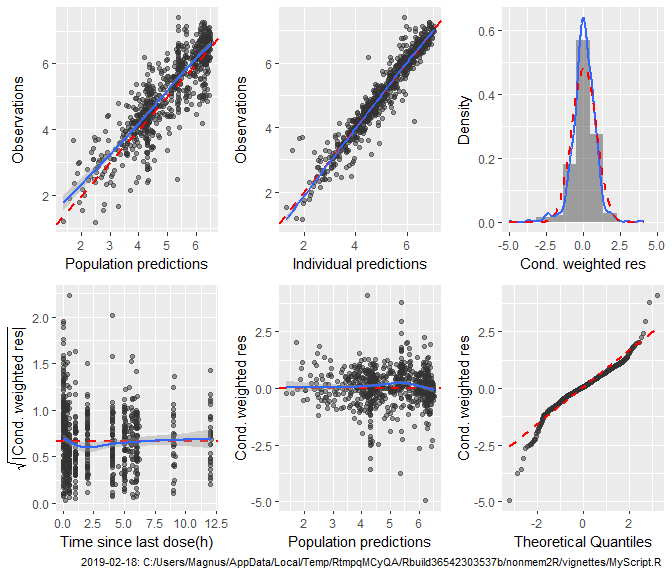There are 4 tailored functions for doing GOF plots for ETA’s: basic.eta.GOF, eta.cov.GOF, eta.cat.GOF, and eta.pairs.GOF. These functions identifies all columns named ETA1,..,ETA9,ET10,.. in the input data.frame and provides different types of GOF plots for these columns. All 4 functions will by default exclude ETA’s which are constant i.e. when set as FIX in your nonmem model (use drop.fixed=FALSE to override) and each ETA is scaled to unit variance (use standardize=FALSE to override). All but the basic.eta.GOF function return an ggplot object and can be further modified as any ggplot object.

basic.eta.GOF will provide GOF plots showing histograms and densities combined with QQ-norm plots.

basic.eta.GOF(sdtab)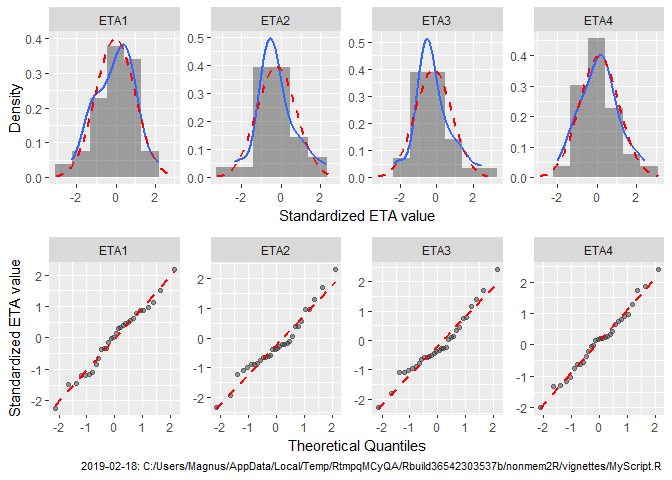eta.cov.GOF will provide GOF plots showing x-y plots of ETA’s vs continuous covariates and eta.cat.GOF will provide box-plots of all ETA’s. Both function have an argument type default set to “all-in-one”. This will generate a ggplot which is formatted by facet_grid with all ETA’s in rows and all covariates in columns, as in the example below for eta.cov.GOF.

eta.cov.GOF(subset(sdtab,DV>0),covariates=c("AGE","BWT"))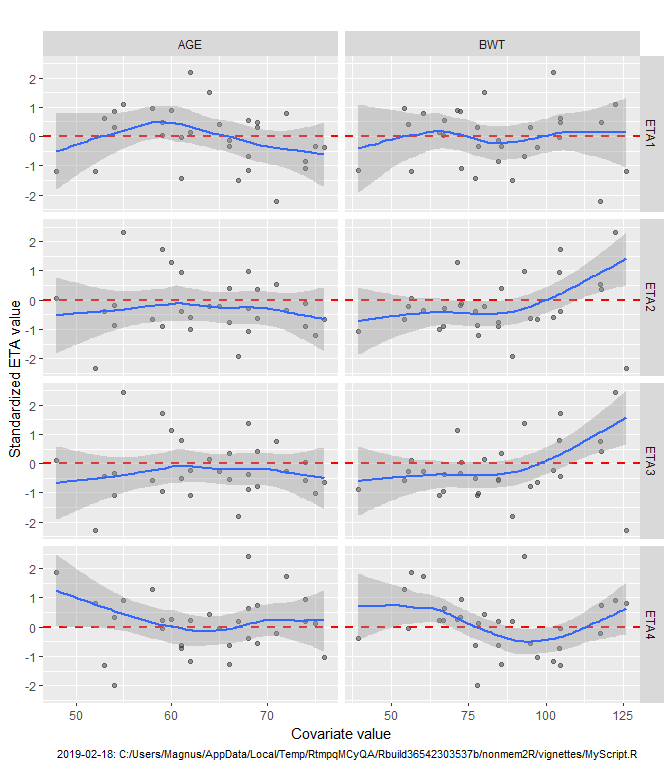However it’s also possible to get either one page for each ETA (type="eta-by-page") or as in the example below for eta.cat.GOF with one page for each covariate (type="covariate-by-page").

eta.cat.GOF(subset(sdtab,DV>0),covariates=c("gender","eGFR"),type="covariate-by-page")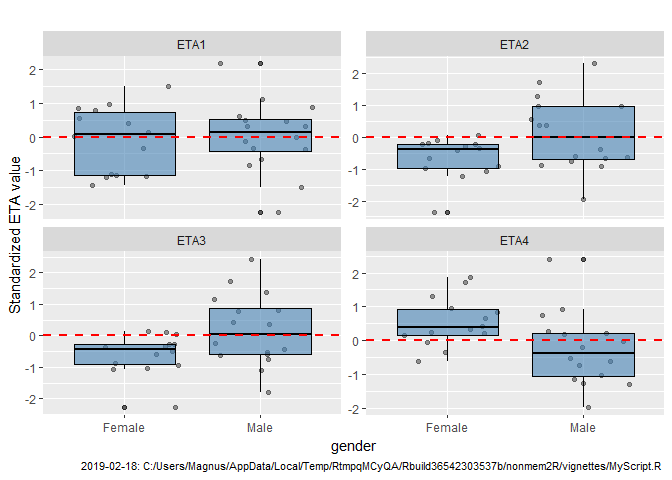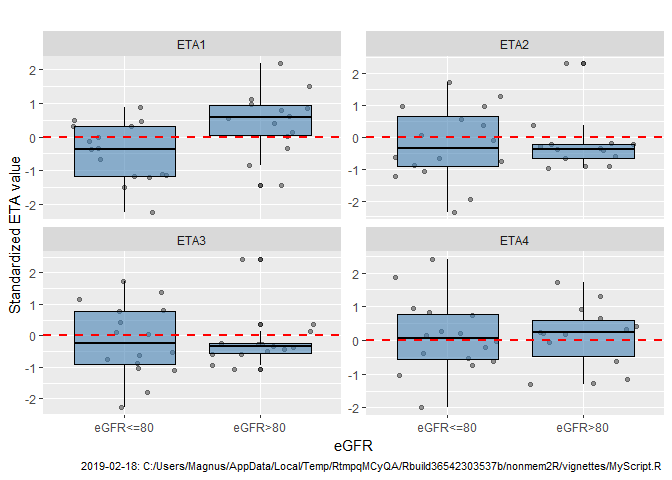The last tailored function for doing GOF plots of ETA’s is eta.pairs.GOF with an example below. Default setting will show scatter plots below the diagonal, smooth density on the diagonal, and spearman correlations above the diagonal. A 2D density can also be added on either side of the diagonal. The example below is show the effect of setting labels for ETA using set.GOF.params

set.GOF.params(eta.labels=c("Ka","Vc","CL","F"))
eta.pairs.GOF(sdtab,density2D="lower")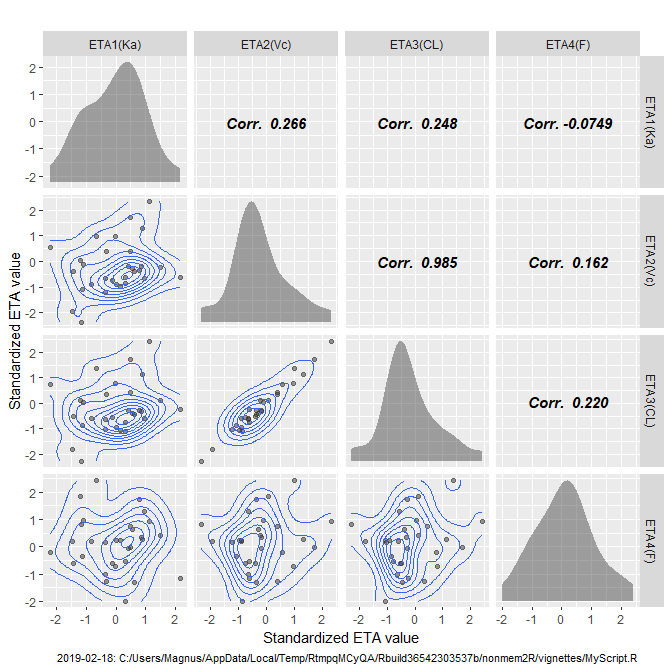As mentioned some of the functions don’t return and ggplot object and the graph can thus not be further modified by adding existing ggplot functions. The functions do however have a named argument global.ggplot.options which is added to each individual ggplot functions before combining. This functionality is limited to one single additional ggplot function, e.g. facet_grid, facet_wrap or different theme’s such as theme_bw.

In the example below basic.GOF4 is modified be setting global.ggplot.options to facet_wrap(~gender) to get one panel for each gender for each of the four GOF plots.

basic.GOF4(subset(sdtab,DV>0),idv2="TAPD",global.ggplot.options=facet_wrap(~gender))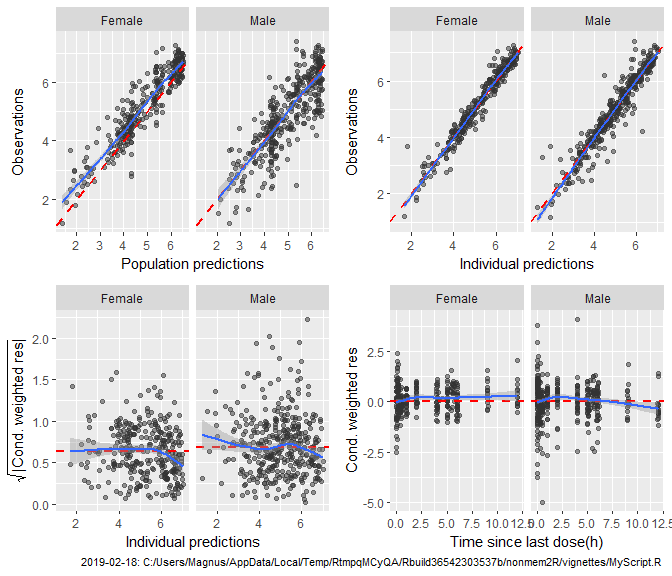And in the example below basic.GOF6 is modified be setting global.ggplot.options to theme_classic()

basic.GOF6(subset(sdtab,DV>0),idv2="TAPD",global.ggplot.options=theme_classic())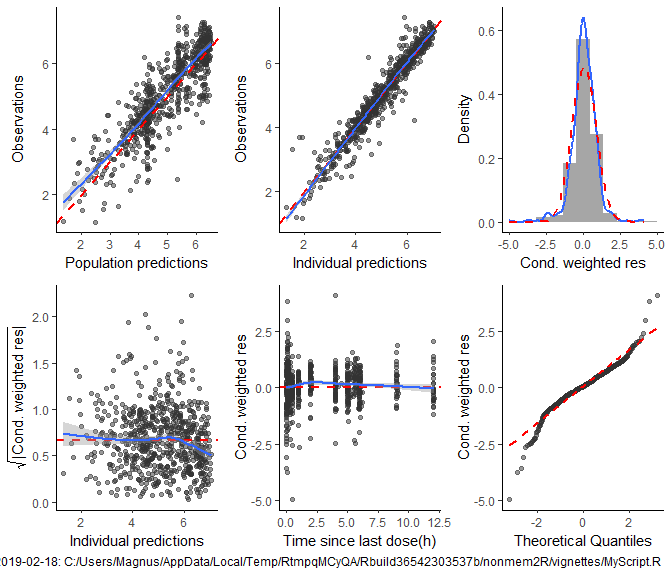### Labels and formatting options

#### Labels

The function get.label is used to set good looking axis labels for the most common NONMEM variables, e.g. CWRES is by default labelled “Cond. weighted res”. The dictionary of labels is controlled by the function set.GOF.dictionary.
In the example below the label for TAD is changed to “Time since last dose(days)” and a new label “Time after first dose (days)” is added for the variable TIME.

set.GOF.dictionary(TAD="Time since last dose(days)")
set.GOF.dictionary(TIME="Time since first dose(days)")

Use get.GOF.dictionary to inspect the complete dictionary of labels and default.GOF.dictionary to reset all labels to the default. It is also possible to turn of functionality and instead have column names as is for axis labels by setting the global GOF parameter axis.labels to FALSE. See next section for how to change global GOF parameters.

get.GOF.dictionary()
## $DV ##  "Observations" ## ##$PRED
##  "Population predictions"
##
## $IPRED ##  "Individual predictions" ## ##$CWRES
##  "Cond. weighted res"
##
## $IWRES ##  "Ind. weighted res" ## ##$TIME
##  "Time since first dose(days)"
##
## $TAPD ##  "Time since last dose(h)" ## ##$TAD
##  "Time since last dose(days)"
##
## $TAFD ##  "Time after first dose(h)" #### Formatting with set.GOF.params The formatting of lines, plot symbols and caption text is controlled by the argument control with default values controlled by the current setting of GOF parameters. A default setting of parameters is set on loading nonmem2R BUT can be modified using set.GOF.params. For example to change plot symbols for one GOF use control=GOF.control(pch.data = 1) to get circles instead of the default which is points (pch=19). To change so that all GOF’s within a script or project get a different formatting, instead use set.GOF.params(pch.data = 1), see below example. set.GOF.params(pch.data = 1) do.one.GOF(subset(sdtab,DV>0),"PRED","DV")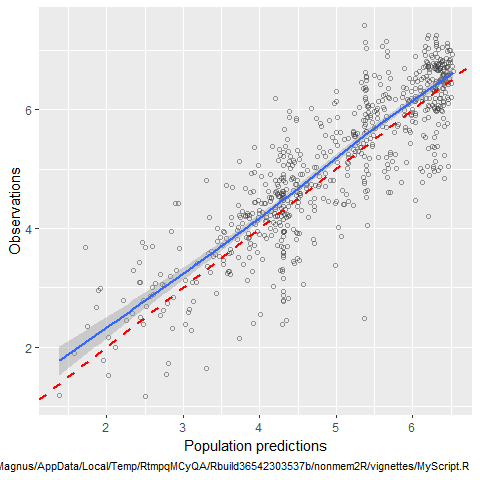The format of the caption can be changed to have a different colour or font size either for only one GOF using the control argument, or for all GOF’s using the set.GOF.params. In the below example the formatting is changed to a larger font size, and the captions only displays a partial path including only 3 folders. (We change back to the default after using basic.GOF4) set.GOF.params(caption.path.depth=3,size.caption = 14,pch.data = 19) basic.GOF4(subset(sdtab,DV>0))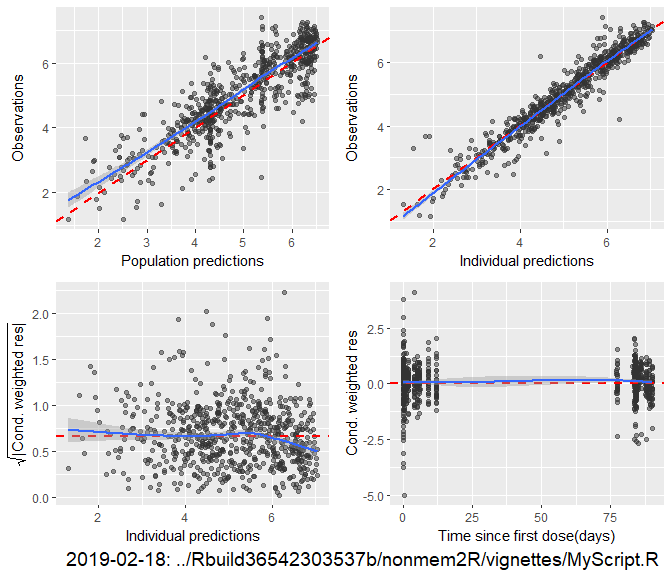### Change back to default set.GOF.params(caption.path.depth=99,size.caption =8) The caption text is set by the function get.caption which also is a GOF parameter that can be modified like any other GOF parameter using set.GOF.params. The default caption as shown in all examples above is a one-row caption with date and full path to the script. In the below example the get.caption function is modified to instead put the path to current working directory, the scripts name, and the date on separate rows. If modifying the function for caption text please not that the get.caption GOF parameter must be just that; a function with no argument returning a character string. set.GOF.params( get.caption=function(x){ paste(getwd(),get.GOF.params()$script.name,date(),sep="\n")
}
)
do.one.GOF(subset(sdtab,DV>0),"PRED","DV")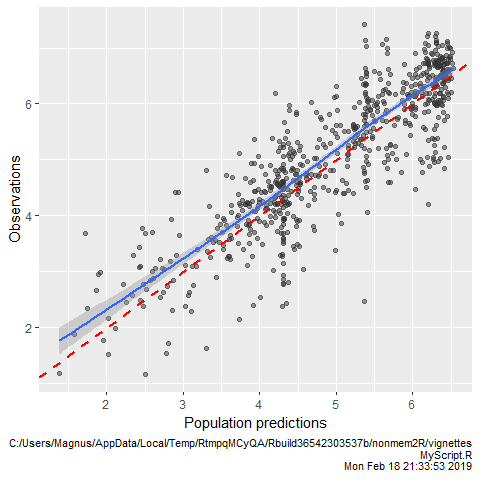Note that there is no check that the new value set for a GOF parameter make sense. To list all GOF parameters use get.GOF.params and default.GOF.params to reset all GOF parameters to the default. See section Details in packages documention for get.GOF.params.

get.GOF.params()
## $col.data ##  "gray20" ## ##$cex.data
##  1.5
##
## $pch.data ##  19 ## ##$alpha.data
##  0.5
##
## $col.smooth ##  "#3366FF" ## ##$lty.smooth
##  1
##
## $lwd.smooth ##  1 ## ##$se.smooth
##  TRUE
##
## $span.smooth ##  0.6666667 ## ##$degree.smooth
##  1
##
## $family.smooth ##  "symmetric" ## ##$col.refline
##  "red"
##
## $lty.refline ##  2 ## ##$lwd.refline
##  1
##
## $fill.hist ##  "gray30" ## ##$col.hist
##  "transparent"
##
## $alpha.hist ##  0.5 ## ##$fill.box
##  "steelblue"
##
## $col.box ##  "black" ## ##$alpha.box
##  0.6
##
## $axis.labels ##  TRUE ## ##$add.caption
##  TRUE
##
## $size.caption ##  8 ## ##$col.caption
##  1
##
## $caption.path.depth ##  99 ## ##$get.caption
## function (x)
## {
##     paste(getwd(), get.GOF.params()$script.name, date(), sep = "\n") ## } ## ##$corr.fontface
##  4
##
## $eta.labels ##  "Ka" "Vc" "CL" "F" ## ##$script.name
##  "MyScript.R"

### GOF building block’s

This set of functions serve as building blocks for creating your own favourite set of graphs. All return an ggplot object and the output can therefore be further modified.

do.one.GOF is a simple x-y plot where you specify variables as strings, a smoother is added by default (blue line) but can be omitted. A red ref-line at y~x (default), or horizontal (at median or mean) can also be added. There are also options for the smoother. In the below example observed data (DV) is plotted versus predictions (PRED) and the graph is stratified by gender by adding the ggplot2 function facet_wrap.

do.one.GOF(subset(sdtab,DV>0),x="PRED",y="DV")+facet_wrap(~gender)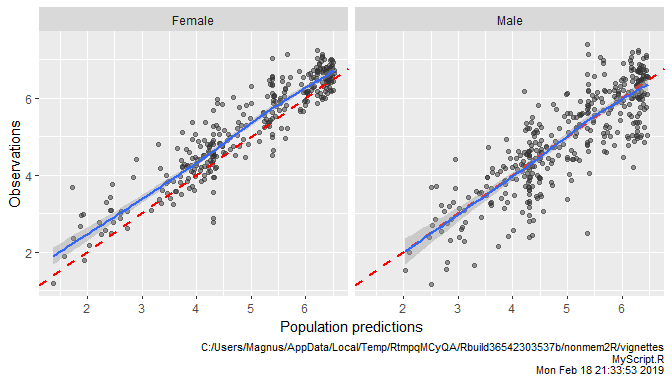do.multi.GOF do multiple y-x plots where x is shared. A smoother is added by default, but reference lines are however omitted but can be added with similar options as for do.one.GOF. Adding additional ggplot functions can be done similar as for do.one.GOF e.g. the ggplot function theme_bw as in the below example. However, since the multi-panel layout is generated by the facet facet_wrap(~variable), if modifying or adding additional facet’s it’s important to keep separate panels by variable and the recommend option is to use facet_grid. For example, adding facet_grid(variable~gender) will provide a do.multi.GOF stratified by gender.

do.multi.GOF(subset(sdtab,DV>0),x="TAPD",y=c("DV","PRED","IPRED"))+theme_bw()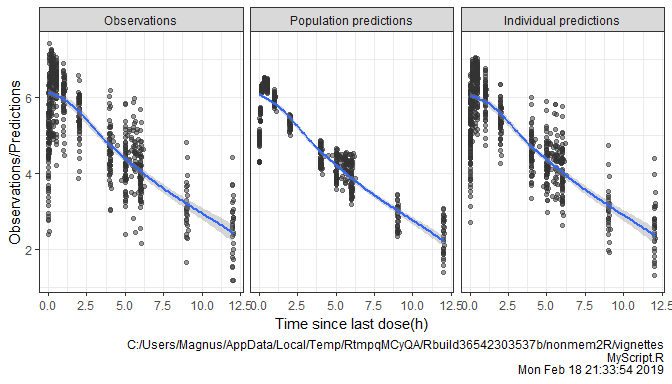histGOF and qqnormGOF provide GOF’s for residuals and ETA’s to check for assumption of normality. As for the other GOF’s red lines are reference lines, blue lines are smoother’s representing the actual data. For histGOF this is a smooth density whereas there is no smoother option for qqnormGOF. We demonstrate these functions by adding extra ggplot functions, in this case facet_wrap to stratify by time after dose into four categories.

sdtab$TAPDgr<-cut(sdtab$TAPD,breaks=c(0,0.5,2,10,25))
histGOF(subset(sdtab,DV>0),"CWRES")+facet_wrap(~TAPDgr,nrow=1)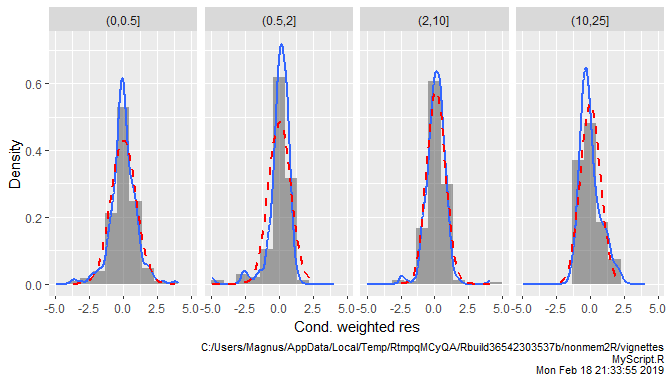qqnormGOF(subset(sdtab,DV>0),"CWRES")+facet_wrap(~TAPDgr,nrow=1)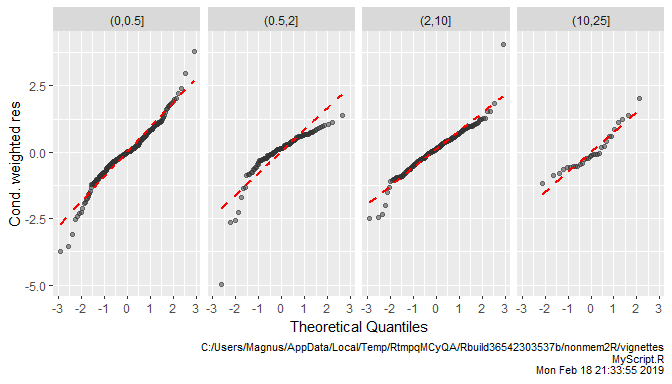eta.hist.GOF and eta.qqnorm.GOF are functions specific for ETA columns in NONMEM output tables. The functions identifies all columns named ETA1,..,ETA9,ET10,... and provide a GOF plot stratified by the ETA columns found. They provide similar type of graphs as histGOF and qqnormGOF. As for do.multi.GOF the function use facet_wrap(~variable). The recommend option to add or modify facet is to use facet_grid. For example, adding facet_grid(gender~variable) will provide a GOF of ETA’s stratified by gender.

eta.qqnorm.GOF(subset(sdtab,DV>0))+facet_grid(gender~variable)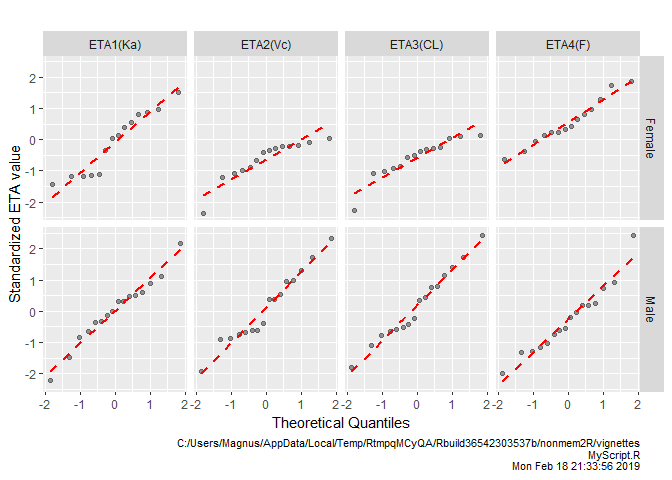### GOF builders

Finally, there are also a set of functions that can be used to combine any type of ggplot objects and plot them in one single graph: merge2GOF, merge4GOF, and merge6GOF. They differ in how many graphs they can combine: 1x2, 2x2, and 2x3, otherwise they share the same set of arguments.

In the example below GOF building blocks and GOF builders are used to generate a graph similar to basic.GOF4.

p1<-do.one.GOF(subset(sdtab,DV>0),
"PRED",
"DV",
p2<-do.one.GOF(subset(sdtab,DV>0),
"IPRED",
"DV",
sqrt.abs<-function(x){sqrt(abs(x))}
p3<-do.one.GOF(subset(sdtab,DV>0),
"IPRED",
"CWRES",
refline="hrefmedian",
fy=sqrt.abs,
merge4GOF(p1,p2,p3,p4)